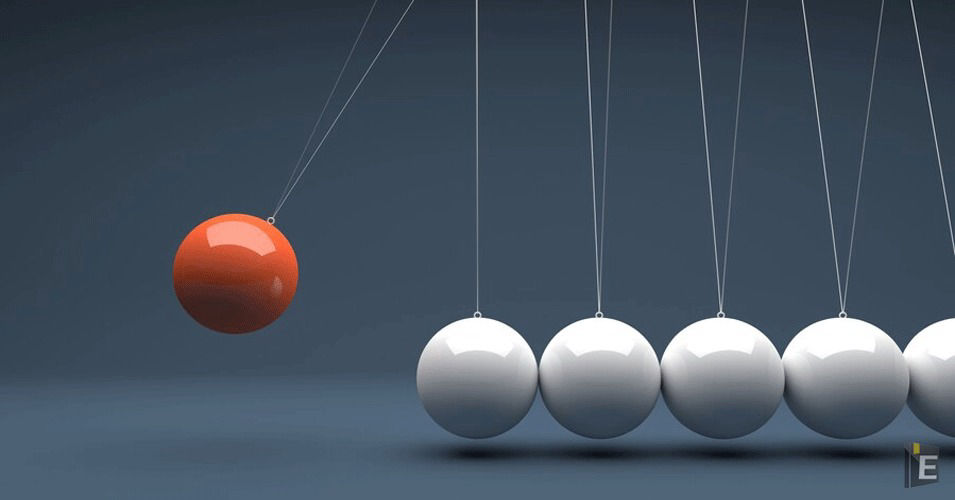• Save

# Physics GK Quiz Questions and AnswersPhysics is the branch of science that deals with the structure of matter and the interactions between the fundamental constituents of the observable universe. It studies natural matter, its fundamental constituents, its motion and behavior through space and time, and the associated entities of energy and force. Questions related to Physics General Knowledge Quiz are asked in competitive exams under the General Science section.

## Physics GK Quiz

Here, I am sharing Physics GK Quiz Questions and Answers related to motion, speed, space and time, energy, force etc under the General Science section for those aspirants who are finding the best Physics GK Questions for upcoming competitive exams. Through this article Physics GK Quiz Questions and Answers, you can do the best practice of Physics GK.

Also, Read Latest Current Affairs Questions 2022: Current Affairs Today

Students can quickly get free General Knowledge Mock Test and Current Affairs Mock Test on this platform for online exam practice to obtain good marks in competitive exams.

## Physics GK Quiz Questions and Answers

Q :

A wooden swing is swinging in a sitting position, the time period of oscillations when the girl is standing?

(A) will decrease

(B) remain unchanged

(C) will be more

(D) none of these

Q :

Skating on ice shows that on increasing the pressure the melting point of ice

(A) decreases

(B) increases

(C) remains unchanged

(D) first decreases then increases

Q :

Which of the following does not have kinetic energy?

(A) fired bullet

(B) drawn bow

(C) moving hammer

(D) flowing water

Q :

When milk is churned, the butter separates from it, what is the reason for this?

(A) Gravitational force

(B) Frictional force

(C) Centrifugal force

(D) Heat

Q :

Which of the following devices converts alternating current into direct current?

(A) Alternator

(B) Condenser

(C) Transformer

(D) None of these

Q :

Which of the following pairs of physical quantities do not have the same dimensional formula?

(A) Force and Pressure

(B) Weight and Force

(C) Impulse and Momentum

(D) Work and Energy

Q :

Ice is floating on water in a beaker when the ice melts completely, the level of water in the beaker will be?

(A) will increase

(B) first increase then decrease

(C) will decrease

(D) will remain the same

Explanation :

Therefore, when the ice melts its volume decreases but the volume of water will remain the same. Hence even after the ice melts, there will be no rise in the level of the water. So, the level of the water will remain unchanged.

Q :

A person climbing a hill leans forward because?

(A) Able to walk fast

(B) To increase stability

(C) To conserve power

(D) Reduce the chances of slipping

Q :

Objects falling freely in void?

(A) have the same velocity

(B) same force

(C) uniform speed

(D) have uniform acceleration

Q :

Two vectors whose values are different?

(A) Their direction will be different

(B) His result will be zero

(C) Their result cannot be zero

(D) None of these